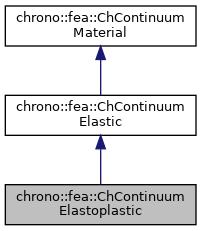chrono::fea::ChContinuumElastoplastic Class Referenceabstract

## Description

Class for all elastic materials that can undergo plastic flow.

Defines simply some interface functions.

#include <ChContinuumMaterial.h>

Inheritance diagram for chrono::fea::ChContinuumElastoplastic:[legend]
Collaboration diagram for chrono::fea::ChContinuumElastoplastic:[legend]

## Public Member Functions

ChContinuumElastoplastic (double myoung=10000000, double mpoisson=0.4, double mdensity=1000)

virtual double ComputeYeldFunction (const ChStressTensor<> &mstress) const =0
Return a scalar value that is 0 on the yield surface, <0 inside (elastic), >0 outside (incompatible->plastic flow)

virtual void ComputePlasticStrainFlow (ChStrainTensor<> &mplasticstrainflow, const ChStrainTensor<> &mtotstrain) const =0
Compute plastic strain flow (flow derivative dE_plast/dt) from strain, according to VonMises strain yield theory.

virtual void ComputeReturnMapping (ChStrainTensor<> &mplasticstrainflow, const ChStrainTensor<> &mincrementstrain, const ChStrainTensor<> &mlastelasticstrain, const ChStrainTensor<> &mlastplasticstrain) const =0
Correct the strain-stress by enforcing that elastic stress must remain on the yield surface, computing a plastic flow to be added to plastic strain while integrating.

virtual void Set_flow_rate (double mflow_rate)=0
Set the plastic flow rate, i.e. More...

virtual double Get_flow_rate () const =0
Set the plastic flow rate.

virtual void ArchiveOUT (ChArchiveOut &marchive) override

virtual void ArchiveIN (ChArchiveIn &marchive) overridePublic Member Functions inherited from chrono::fea::ChContinuumElastic
ChContinuumElastic (double myoung=10000000, double mpoisson=0.4, double mdensity=1000)
Create a continuum isotropic hookean material. More...

ChContinuumElastic (const ChContinuumElastic &other)

void Set_E (double m_E)
Set the Young E elastic modulus, in Pa (N/m^2), as the ratio of the uniaxial stress over the uniaxial strain, for hookean materials. More...

double Get_E () const
Get the Young E elastic modulus, in Pa (N/m^2).

void Set_v (double m_v)
Set the Poisson v ratio, as v=-transverse_strain/axial_strain, so takes into account the 'squeezing' effect of materials that are pulled (so, if zero, when you push the two sizes of a cube, it won't inflate). More...

double Get_v () const
Get the Young v ratio, as v=-transverse_strain/axial_strain.

void Set_G (double m_G)
Set the shear modulus G, in Pa (N/m^2), as the ratio of shear stress to the shear strain. More...

double Get_G () const
Get the shear modulus G, in Pa (N/m^2)

double Get_l () const
Get Lamé first parameter (the second is shear modulus, so Get_G() )

double Get_BulkModulus () const
Get bulk modulus (increase of pressure for decrease of volume), in Pa.

double Get_WaveModulus () const
Get P-wave modulus (if V=speed of propagation of a P-wave, then (M/density)=V^2 )

void ComputeStressStrainMatrix ()
Computes Elasticity matrix and stores the value in this->StressStrainMatrix Note: is performed every time you change a material parameter.

ChMatrixDynamicGet_StressStrainMatrix ()
Get the Elasticity matrix.

void ComputeElasticStress (ChStressTensor<> &mstress, const ChStrainTensor<> &mstrain) const
Compute elastic stress from elastic strain (using column tensors, in Voight notation)

void ComputeElasticStrain (ChStrainTensor<> &mstrain, const ChStressTensor<> &mstress) const
Compute elastic strain from elastic stress (using column tensors, in Voight notation)

void Set_RayleighDampingM (double m_d)
Set the Rayleigh mass-proportional damping factor alpha, to build damping R as R=alpha*M + beta*K.

double Get_RayleighDampingM () const
Set the Rayleigh mass-proportional damping factor alpha, in R=alpha*M + beta*K.

void Set_RayleighDampingK (double m_d)
Set the Rayleigh stiffness-proportional damping factor beta, to build damping R as R=alpha*M + beta*K.

double Get_RayleighDampingK () const
Set the Rayleigh stiffness-proportional damping factor beta, in R=alpha*M + beta*K.Public Member Functions inherited from chrono::fea::ChContinuumMaterial
ChContinuumMaterial (double mdensity=1000)

ChContinuumMaterial (const ChContinuumMaterial &other)

void Set_density (double m_density)
Set the density of the material, in kg/m^2.

double Get_density () const
Get the density of the material, in kg/m^2.Protected Attributes inherited from chrono::fea::ChContinuumMaterial
double density

## ◆ Set_flow_rate()

 virtual void chrono::fea::ChContinuumElastoplastic::Set_flow_rate ( double mflow_rate )
pure virtual

Set the plastic flow rate, i.e.

the 'creep' speed. The lower the value, the slower the plastic flow during dynamic simulations, with delayed plasticity.

Implemented in chrono::fea::ChContinuumDruckerPrager, and chrono::fea::ChContinuumPlasticVonMises.

The documentation for this class was generated from the following files:
• /builds/uwsbel/chrono/src/chrono/fea/ChContinuumMaterial.h
• /builds/uwsbel/chrono/src/chrono/fea/ChContinuumMaterial.cpp